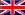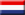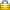# Check Dutch bank account number or citizen service number with Eleven test

Select language:Elfproef (Dutch for Eleven test or 11 test) is a test that is used to validate Dutch bank account numbers.
It is a rudimentary check to validate the correctness of the bank account number.

It only applies to bank account numbers with 9 or 10 digits. Dutch bank account numbers have maximum 10 digits. Dutch bank account numbers with 8 digits does not exist. The Eleven test is not applied on account numbers with 1 till 7 digits. These account numbers are called "Girorekeningnummers" and are only used by the Dutch bank Postbank. In 2009 Postbank merged with ING Bank and is now called ING. These "Girorekeningnummers" are still in use.

The Eleven test is also used to validate Dutch citizen service number (Burgerservicenummer or BSN, formerly known as SoFinummer). The Dutch citizen service number is a unique personal ID number, and every Dutch citizen who is registered in the population register at the municipal authorities has one. This citizen service number is used by all Dutch government organisations. The Dutch citizen service number has always 9 digits and the first number can be a 0.

Example 1:

How the Eleven test is applied on a Dutch bank account number with 9 digits: 755490975
``` 1st number of the bank account number = 7    7 * 9 =  63
2nd number of the bank account number = 5    5 * 8 =  40
3rd number of the bank account number = 5    5 * 7 =  35
4th number of the bank account number = 4    4 * 6 =  24
5th number of the bank account number = 9    9 * 5 =  45
6th number of the bank account number = 0    0 * 4 =   0
7th number of the bank account number = 9    9 * 3 =  27
8th number of the bank account number = 7    7 * 2 =  14
9th number of the bank account number = 5    5 * 1 =   5
========================================================
Sum = 253
Eleven test: 253 modulo 11 = 0
Bank account number: 755490975 is correct!
```
Example 2:
How the Eleven test is applied on a Dutch bank account number with 10 digits: 7584955151
``` 1st  number of the bank account number = 7    7 * 10 =  70
2nd  number of the bank account number = 5    5 *  9 =  45
3rd  number of the bank account number = 8    8 *  8 =  64
4th  number of the bank account number = 4    4 *  7 =  28
5th  number of the bank account number = 9    9 *  6 =  54
6th  number of the bank account number = 5    5 *  5 =  25
7th  number of the bank account number = 5    5 *  4 =  20
8th  number of the bank account number = 1    1 *  3 =   3
9th  number of the bank account number = 5    5 *  2 =  10
10th number of the bank account number = 1    1 *  1 =   1
==========================================================
Sum = 320
Eleven test: 320 modulo 11 = 1
Bank account number: 7584955151 is not correct! Modulo must be 0.
```
Example 3:
How the Eleven test is applied on a Dutch citizen service number (Burgerservicenummer) with 9 digits: 224333264
``` 1st number of the citizen service number = 2    2 * 9 =  18
2nd number of the citizen service number = 2    2 * 8 =  16
3rd number of the citizen service number = 4    4 * 7 =  28
4th number of the citizen service number = 3    3 * 6 =  18
5th number of the citizen service number = 3    3 * 5 =  15
6th number of the citizen service number = 3    3 * 4 =  12
7th number of the citizen service number = 2    2 * 3 =   6
8th number of the citizen service number = 6    6 * 2 =  12
9th number of the citizen service number = 4    4 *-1 =  -4 <== Multiply with -1
===========================================================
Sum = 121
Eleven test: 121 modulo 11 = 0
Citizen service number: 224333264 is correct!
```
With this service you can:
• Test one or more Dutch bank account numbers if they comply with the Eleven test.
• Test one or more Dutch citizen service numbers if they comply with the Eleven test.
All information entered in the input fields marked with the key iconare securely send to Mobilefish.com.

YOU USE THIS SERVICE AT YOUR OWN RISK. MOBILEFISH.COM WILL NOT BE LIABLE FOR ANY DATA LOSS, DAMAGES, LOSS OF PROFITS OR ANY OTHER KIND OF LOSS WHILE USING OR MISUSING THIS SERVICE.

## Input Eleven test:

 The numbers are *:Dutch bank account numbers Dutch citizen service numbersEnter the numbers each on a separate line. Max. 100000 numbers. *:To prevent automated submissions an Access Code has been implemented for this tool.Please enter the Access Code as displayed above. *: * = required